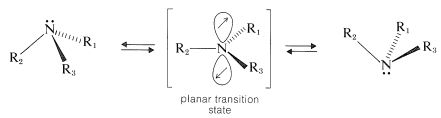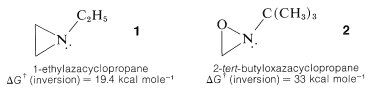# 23.6: Stereochemistry of Amines

$$\newcommand{\vecs}{\overset { \rightharpoonup} {\mathbf{#1}} }$$ $$\newcommand{\vecd}{\overset{-\!-\!\rightharpoonup}{\vphantom{a}\smash {#1}}}$$$$\newcommand{\id}{\mathrm{id}}$$ $$\newcommand{\Span}{\mathrm{span}}$$ $$\newcommand{\kernel}{\mathrm{null}\,}$$ $$\newcommand{\range}{\mathrm{range}\,}$$ $$\newcommand{\RealPart}{\mathrm{Re}}$$ $$\newcommand{\ImaginaryPart}{\mathrm{Im}}$$ $$\newcommand{\Argument}{\mathrm{Arg}}$$ $$\newcommand{\norm}{\| #1 \|}$$ $$\newcommand{\inner}{\langle #1, #2 \rangle}$$ $$\newcommand{\Span}{\mathrm{span}}$$ $$\newcommand{\id}{\mathrm{id}}$$ $$\newcommand{\Span}{\mathrm{span}}$$ $$\newcommand{\kernel}{\mathrm{null}\,}$$ $$\newcommand{\range}{\mathrm{range}\,}$$ $$\newcommand{\RealPart}{\mathrm{Re}}$$ $$\newcommand{\ImaginaryPart}{\mathrm{Im}}$$ $$\newcommand{\Argument}{\mathrm{Arg}}$$ $$\newcommand{\norm}{\| #1 \|}$$ $$\newcommand{\inner}{\langle #1, #2 \rangle}$$ $$\newcommand{\Span}{\mathrm{span}}$$$$\newcommand{\AA}{\unicode[.8,0]{x212B}}$$

In ammonia and amines, the bonds to nitrogen are pyramidal with bond angles closer to the tetrahedral value of $$109.5^\text{o}$$ than to the $$90^\text{o}$$ value expected for the use of pure $$p$$ orbitals of nitrogen in bond formation. We consider that the nitrogen in amines is formulated best with hybrid $$sp^3$$-type orbitals; three of these orbitals are used in $$\sigma$$-bond formation while the fourth contains the nonbonding electron pair:A consequence of the pyramidal configuration at nitrogen is that, when the attached groups $$\ce{R}_1$$, $$\ce{R}_2$$, and $$\ce{R}_3$$ are nonidentical, the nitrogen becomes a chiral atom. Under these circumstances, we would expect two enantiomeric configurations:The resolution of an acyclic chiral amine into its separate enantiomers has not been achieved yet, and it appears that the enantiomers are very rapidly interconverted by an inversion process involving a planar transition state:With ammonia, inversion of this type occurs about $$4 \times 10^{10}$$ times per second at room temperature, which corresponds to the planar state being less stable than the pyramidal state by about $$6 \: \text{kcal mol}^{-1}$$. With aliphatic tertiary amines, the inversion rate is more on the order of $$10^3$$ to $$10^5$$ times per second. Such rates of inversion are much to great to permit resolution of an amine into its enantiomers by presently available techniques.

When the amine nitrogen is incorporated in a small ring, as in azacyclopropanes, $$1$$, the rate of inversion at nitrogen is markedly slower than in open-chain amines. In fact, with some oxazacyclopropanes, such as $$2$$, inversion does not occur rapidly at ordinary temperatures, which means that the configuration at the nitrogen persists long enough for resolution into enantiomers to be possible:The stereochemistry of azacyclohexanes is complicated by the fact that there is a conformational change in the ring as well as inversion at the pyramidal nitrogen. Therefore it is difficult to say whether the axial-equatorial equilibrium of, for example, 1-methylazacyclohexane is achieved by ring inversion, or by nitrogen inversion, or both: